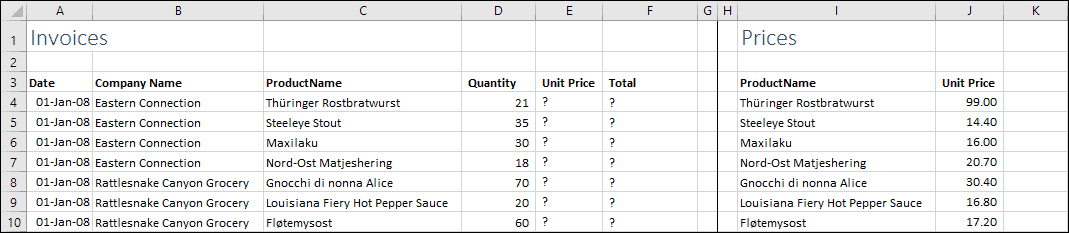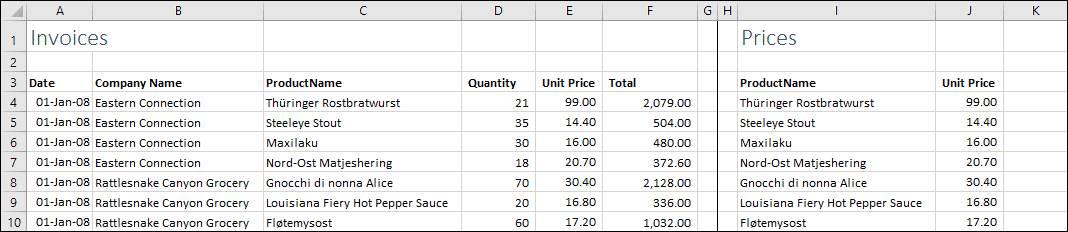# VLOOKUP using text strings

## What is VLOOKUP?

VLOOKUP is one of the functions in Excel’s vast function library. Its purpose is to search a table of values for a corresponding item and return a matching item from the table.

An example might be searching a table of price data for a certain product name and returning the matching product’s price.If you’re unfamiliar with Excel functions, you might want to work through our completely free Basic Skills course, which explains the basics of Excel formulas and functions.

## What is VLOOKUP useful for?

VLOOKUP is generally used when you have two tables of data and need to extract values from one into the other, as shown above.

In the example above you want to extract the price for each product into column E. To do this you would use VLOOKUP to search for the product name in the table of prices and return the Unit Price for the matching product.

## Can VLOOKUP work with text as well as numbers?

Yes. VLOOKUP can search for textual values just as well as it can search for numbers. The example above would search for the product names, which are text rather than numbers.

Remember, of course, that the values being searched should be unique. VLOOKUP stops searching when it finds a single matching item, so it won’t find any further matches if they exist. If there were two entries for a product in the Prices table, VLOOKUP would only return the first value.

## How to use VLOOKUP

Let’s look at the example again:In cell D1 you need to search for the price of Thuringer Rostbratwurst in the Prices table (cells I4:J88) and return the matching price. The VLOOKUP formula to do this is:

=VLOOKUP(C4,I4:J88,2,FALSE)

The formula’s arguments (the values in brackets) work like this:

1. Cell C4 contains the value you are searching for.
2. Cells I4:J88 contain the data that needs to be searched. VLOOKUP always looks in the first column of this data (column I) for the lookup value.
3. You want VLOOKUP to return the value from column 2 of the table (the prices).
4. You only want VLOOKUP to return a value if it finds an exact match, so the last argument is FALSE.

VLOOKUP is explained in much more depth in our Expert Skills course, which also explains how to use inexact VLOOKUP functions to return the nearest match.

After filling down the VLOOKUP formula you can multiply the quantity by the price to extract the total.You can download a copy of the workbook showing the formulas in action.

## What isn't VLOOKUP useful for?

It’s important to remember that Excel was designed to be a spreadsheet tool rather than a database. VLOOKUP allows you to make Excel behave in a similar way to database software, but without many of the features of a ‘real’ database.

If you need to create a complex database you should use software that is made for that purpose such as SQL Server, Access or MySQL. You can then use Excel to connect to that database and analyze the data.

That said, Excel 2016 has incorporated many new features to make it easier to work with relational data. The new OLAP and Get & Transform tools allow you to create relationships between Excel tables and query databases in complex ways. Our Expert Skills course explains how to use these, as well as explaining relationships and databases in much more depth.

Scroll to Top